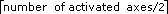# Vector Spaces

NI-Motion Help

Edition Date: August 2012

Part Number: 372134F-01

»View Product InfoDownload Help (Windows Only)

Vector spaces are logical, multidimensional groups of axes. They can be either one-dimensional, two-dimensional with x and y axes, or three-dimensional with x, y, and z axes. The total number of vector spaces NI-Motion supports in your motion control system can be calculated using the following formula:

total vector spaces =.

Vector spaces facilitate 2D and 3D interpolated moves: linear, circular, helical, and spherical. You can send a vector space to many NI-Motion functions to define vector position, vector velocity, vector acceleration, and so on.

Vector spaces are started, stopped, and controlled as if they were a single axis, greatly simplifying the control of coordinated vector axes. All axes in a vector space start and stop at the same time, completing the vector motion profiles programmed. The following table lists the resource IDs and constants for vector space control.

Resource Name Resource ID Constant
Vector Space Control 0x10 NIMC_VECTOR_SPACE_CTRL
Vector Space 1 0x11 NIMC_VECTOR_SPACE1
Vector Space 2 0x12 NIMC_VECTOR_SPACE2
Vector Space 3 0x13 NIMC_VECTOR_SPACE3
Vector Space 4 0x14 NIMC_VECTOR_SPACE4

Functions that can operate on multiple vector spaces simultaneously (for example, Start Motion) can take the vector space control (NIMC_VECTOR_SPACE_CTRL) as their resource parameter.

Vector spaces are configured by mapping axes to the vector space with the Configure Vector Space function. Vector spaces are logical, not physical, and do not require motion resources other than those used by the axes themselves.